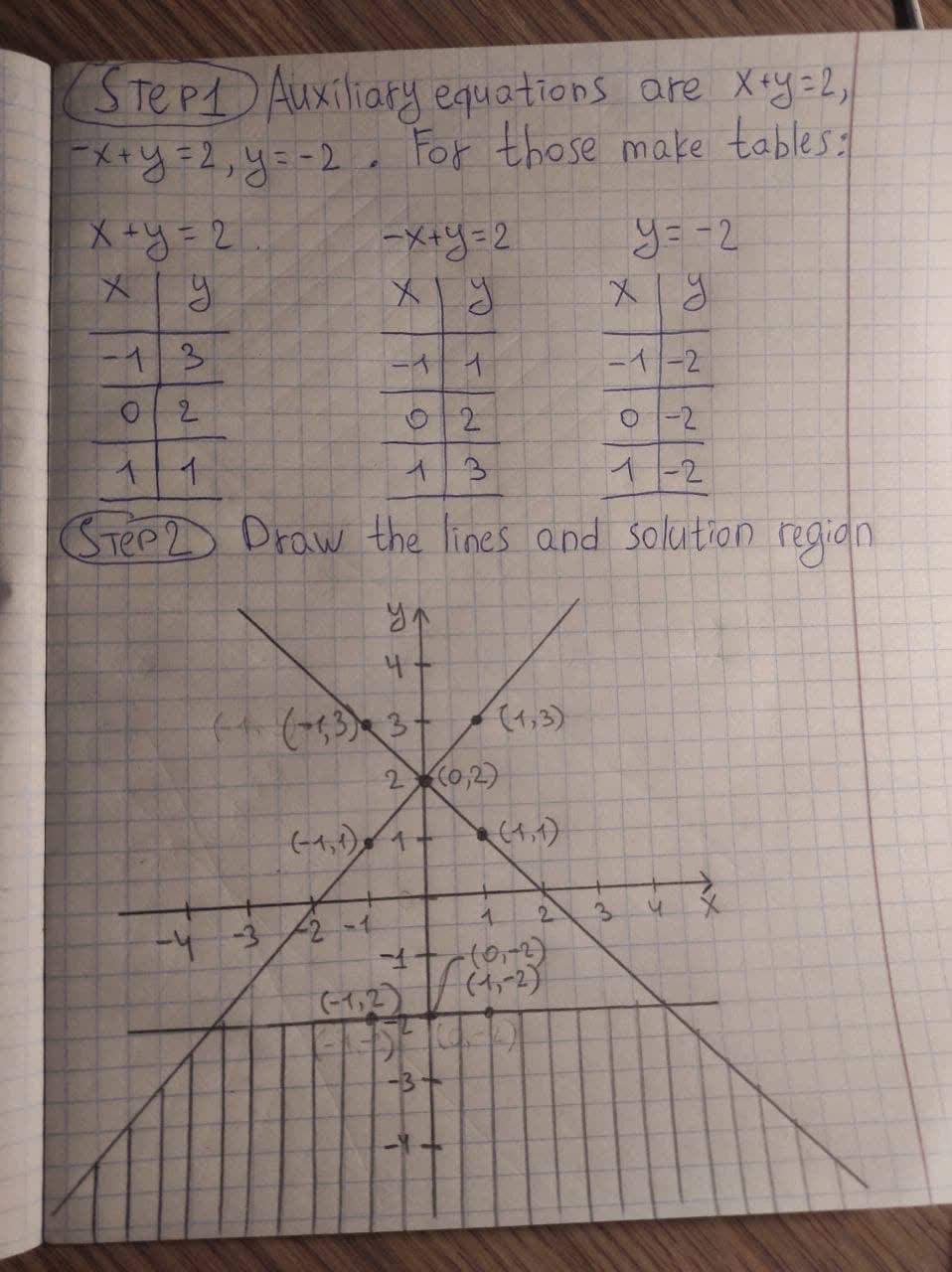# Graph the solution set for the of linear inequalities x+y<=2-x+y<=2y<=-2kuCAu 2021-01-19 Answered

Graph the solution set for the of linear inequalities

$x+y\le 2$

$-x+y\le 2$

$y\le -2$

You can still ask an expert for help

## Want to know more about Inequalities systems and graphs?

• Questions are typically answered in as fast as 30 minutes

Solve your problem for the price of one coffee

• Math expert for every subject
• Pay only if we can solve itwheezym
Step1 Auxiliary equations are x+y=2 , -x+y=2 ,y=-2 For those make tables. Step2 Then draw the lines using the tables. Plot the points and join using lines. Then shade according to the inequalities. Since all inequalities hold equalities so all lines are solid lines. Every inequality is less than equal to. So shade downwards for all. Answer: Solution region (shaded in black)